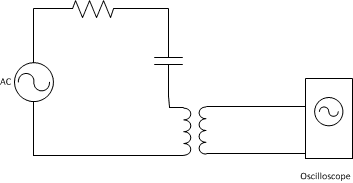# Setting up an LRC series circuit with coupled coils

## Homework Statement

I have been trying to set up an LRC series circuit where in place of an inductor we have a pair of coupled coils. My aim is to determine the resonant frequency in the circuit when the voltage across the resistor or the current in the circuit is at a maximum. I am using a function generator to create a sinusoidal function through the first coil which in turn will create an emf through the second coil placed inside the first. I am then trying to display the resulting waveform on an oscilloscope display along with the input. I am using the second coils dimensions to calculate a theoretical inductor value but I am having trouble correctly connecting the circuit up.

I have connected what I thought was correct but am confused as to how to incorporate the ground from the function generator. Normally I would connect the resistor to the negative (ground) output of the function generator but how do I do that with the coupled coils present?